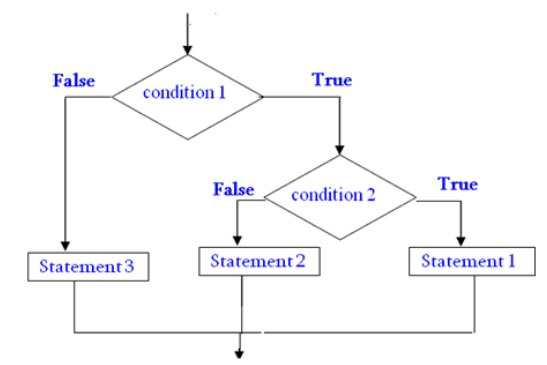Home | | Problem Solving and Python Programming | Conditionals - Python

# Conditionals - Python

Conditional if , Alternative if… else, Chained if…elif…else, Nested if….else

CONDITIONALS

v       Conditional if

v       Alternative if… else

v       Chained if…elif…else

v       Nested if….else

## Conditional (if):

conditional (if) is used to test a condition, if the condition is true the statements inside if will be executed.

syntax:

if(condition 1):

Statement 1

Flowchart:Example:

1.              Program to provide flat rs 500, if the purchase amount is greater than 2000.

2.              Program to provide bonus mark if the category is sports.

### Program to provide flat rs 500, if the purchase amount is greater than 2000.

if(purchase>=2000):

purchase=purchase-500

print(“amount to pay”,purchase)

output

enter   your   purchase

amount

2500

amount to pay

2000

### Program to provide bonus mark if the category is sports

m=eval(input(“enter ur mark out of 100”))

c=input(“enter ur categery G/S”)

if(c==”S”):

m=m+5

print(“mark is”,m)

output

enter ur mark out of 100

85

enter ur categery G/S

S

mark is 90

## alternative (if-else)

In the alternative the condition must be true or false. In this else statement can be combined with if statement. The else statement contains the block of code that executes when the condition is false. If the condition is true statements inside the if get executed otherwise else part gets executed. The alternatives are called branches, because they are branches in the flow of execution.

syntax:Flowchart:Examples:

1.              odd or even number

2.              positive or negative number

3.              leap year or not

4. greatest of two numbers

5. eligibility for voting

### Odd or even number

n=eval(input("enter a number"))

if(n%2==0):

print("even number")

else:

print("odd number")

Output

enter a number4

even number

### positive or negative number

n=eval(input("enter a number"))

if(n>=0):

print("positive number")

else:

print("negative number")

Output

enter a number8

positive number

leap year or not

y=eval(input("enter a yaer"))

if(y%4==0):

print("leap year")

else:

print("not leap year")

Output

enter a yaer2000

leap year

### greatest of two numbers

a=eval(input("enter a value:"))

b=eval(input("enter b value:"))

if(a>b):

print("greatest:",a)

else:

print("greatest:",b)

Output

enter a value:4

enter b value:7

greatest: 7

### eligibility for voting

age=eval(input("enter ur age:"))

if(age>=18):

print("you are eligible for vote")

else:

print("you are eligible for vote")

Output

enter ur age:78

you are eligible for vote

## Chained conditionals(if-elif-else)

·                 The elif is short for else if.

·                 This is used to check more than one condition.

·                 If the condition1 is False, it checks the condition2 of the elif block. If all the conditions are False, then the else part is executed.

·                 Among the several if...elif...else part, only one part is executed according to the condition.

•  The if block can have only one else block. But it can have multiple elif blocks.

• The way to express a computation like that is a chained conditional.

syntax:Flowchart:Example:

1.              student mark system

2.              traffic light system

3.              compare two numbers

### student mark system

mark=eval(input("enter ur mark:"))

if(mark>=90):

elif(mark>=80):

elif(mark>=70):

elif(mark>=50):

else:

print("fail")

Output

enter ur mark:78

### traffic light system

colour=input("enter colour of light:")

if(colour=="green"):

print("GO")

elif(colour=="yellow"):

else:

print("STOP")

Output

enter colour of light:green

GO

### compare two numbers

x=eval(input("enter x value:"))

y=eval(input("enter y value:"))

if(x == y):

print("x and y are equal")

elif(x < y):

print("x is less than y")

else:

print("x is greater than y")

Output

enter x value:5

enter y value:7

x is less than y

a=eval(input("enter a value:"))

b=eval(input("enter b value:"))

c=eval(input("enter c value:"))

d=(b*b-4*a*c)

if(d==0):

print("same and real roots")

elif(d>0):

print("diffrent real roots")

else:

print("imaginagry roots")

output

enter a value:1

enter b value:0

enter c value:0

same and real roots

## Nested conditionals

One conditional can also be nested within another. Any number of condition can be nested inside one another. In this, if the condition is true it checks another if condition1. If both the conditions are true statement1 get executed otherwise statement2 get execute. if the condition is false statement3 gets executed

Syntax:Flowchart:Exampe:

1. greatest of three numbers

2. positive negative or zero

## greatest of three numbers

a=eval(input(“enter the value of a”))

b=eval(input(“enter the value of b”))

c=eval(input(“enter the value of c”))

if(a>b):

if(a>c):

print(“the greatest no is”,a)

else:

print(“the greatest no is”,c)

else:

if(b>c):

print(“the greatest no is”,b)

else:

print(“the greatest no is”,c)

### output

enter the value of a 9

enter the value of a 1

enter the value of a 8

the greatest no is 9

## positive negative or zero

n=eval(input("enter the value of n:"))

if(n==0):

print("the number is zero")

else:

if(n>0):

print("the number is positive")

else:

print("the number is negative")

output

enter the value of n:-9

the number is negative

Tags : Python Programming , Problem Solving and Python Programming : Control Flow, Functions
Study Material, Lecturing Notes, Assignment, Reference, Wiki description explanation, brief detail
Problem Solving and Python Programming : Control Flow, Functions : Conditionals - Python | Python Programming